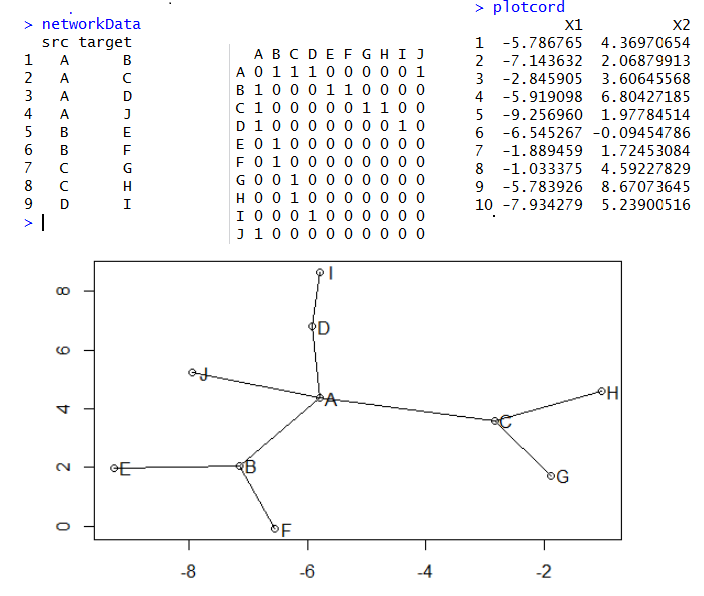R语言画网络图三部曲之sna

plot(plotcord)
text(x=plotcord\$X1+0.2,y=plotcord\$X2,labels = LETTERS[1:10])
for (i in 1:10){
for (j in 1:10){
if(tmp[i,j]) lines(plotcord[c language="(i,j),1"][/c],plotcord[c language="(i,j),2"][/c])
}
}net=network(150, directed=FALSE, density=0.03)
m <- as.matrix.network.adjacency(net) # get sociomatrix
# get coordinates from Fruchterman and Reingold's force-directed placement algorithm.
plotcord <- data.frame(gplot.layout.fruchtermanreingold(m, NULL))
# or get it them from Kamada-Kawai's algorithm:
colnames(plotcord) = c("X1","X2")  ###所有点的坐标，共150个点
edglist <- as.matrix.network.edgelist(net) ##所有点之间的关系-edge ##共335条线
edges <- data.frame(plotcord[edglist[,1],], plotcord[edglist[,2],]) ##两点之间的连线的具体坐标，335条线的起始终止点点坐标

library(network)
library(ggplot2)
library(sna)
library(ergm)